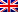# Semi-automatic data analysis

Exploratory data analysis, also known as EDA, has become an increasingly hot topic in data science. As the name suggests, it is a process of trial and error in an uncertain space, with the goal of finding information. This usually happens early in the data science life cycle. In this page, I present a semi-automated EDA (semi-automated data analysis) process.Contenus

## Semi-automatic data analysis: knowing your data

I will use four main libraries: Numpy — for working with arrays; Pandas – to manipulate data in a spreadsheet format we know; Seaborn and matplotlib — to create a data visualization.

`import pandas as pd import seaborn as sns import matplotlib.pyplot as plt import numpy as np from pandas.api.types import is_string_dtype, is_numeric_dtype`

Create a dataframe from the imported dataset by copying the dataset path and use df.head(5) to take a look at the first 5 rows of data.Before we zoom in on each field, let's first look at the general characteristics of the dataset. info() gives the number of non-null values for each column and its data type.describe() provides basic statistics about each column. By passing the 'include='all' parameter, it outputs the count of values, unique count, upper frequency value of categorical variables and count, mean, standard deviation, min, max and percentile of numeric variables.## Missing value and preprocessing

Regarding the subject of missing values, I invite you to choose the tab Correlation and Regressions in the rubric Data Analysis.

## Univariate analysis

The describe() function mentioned in the first section has already provided univariate analysis in a non-graphical way. In this section, we will generate more insights by visualizing the data and spot hidden patterns through graphical analysis.

Categorical variables → Histograms

The easiest and most intuitive way to visualize the property of a categorical variable is to use a bar chart to plot the frequency of each categorical value.

Quantitative variables → Histograms

To graphically represent the distribution of numerical variables, we can use a histogram which is very similar to a bar chart. It divides continuous numbers into groups of equal size and plots the frequency of records between the interval.

I use this for loop to loop through the columns of the data frame and create a plot for each column. Then use a histogram if they are numeric variables and a bar chart if they are categorical variables.## Multivariate analysis: quantitative vs. quantitative

A very important part of semi-automatic data analysis is multivariate analysis, how the columns influence each other.

First, let's use the correlation matrix to find the correlation of all numeric data type columns. Then use a heat map to visualize the result. The annotation inside each cell indicates the correlation coefficient of the relationship.Second, since the correlation matrix only indicates the strength of the linear relationship, it is best to plot the numeric variables using the seaborn sns.pairplot() function. Note that the sns.heatmap() and sns.pairplot() functions ignore non-numeric data types.Here is an example with another dataset:The pair plot or scatterplot is a good complement to the correlation matrix, especially where non-linear relationships (e.g., exponential, inverse relationship) may exist. For example, the inverse relationship between “Rank” and “Sales” seen in the restaurant dataset can be mistaken for a strong linear relationship if we just look at the number “-0.92” in the correlation matrix.

## Category vs Category

The relationship between two categorical variables can be visualized using clustered histograms. The frequency of primary categorical variables is broken down by secondary category. This can be achieved using sns.countplot().

I'm using a nested for loop, where the outer loop loops through all the categorical variables and assigns them as the primary category, then the inner loop loops through the list again to associate the primary category with another secondary category.In a clustered bar chart, if the frequency distribution always follows the same pattern in different clusters, it suggests that there is no dependence between the primary category and the secondary category. However, if the distribution is different, it indicates that there is likely a dependency between two variables.## Category vs Quantitative

The boxplot is usually adopted when we need to compare the variation of numerical data between groups. This is an intuitive way to graphically represent whether the variation in categorical characteristics contributes to the difference in values, which can further be quantified using ANOVA analysis.

In this process, I associate each column of the categorical list with all the columns of the numeric list and plot the boxplot accordingly.In the “reddit_wsb” dataset, no significant differences are observed between the different categories.Let's see the differences that may exist using another dataset.Another approach is based on the pairplot we did earlier for numeric versus numeric. To introduce the categorical variable, we can use different hues to represent. Just like what we did for countplot. To do this, we can simply iterate over the categorical list and add each element as a tint of the pairplot.Here are the results on the second dataset:This marks the end of our semi-automatic data analysis that you can use for all your datasets.FRFRENES